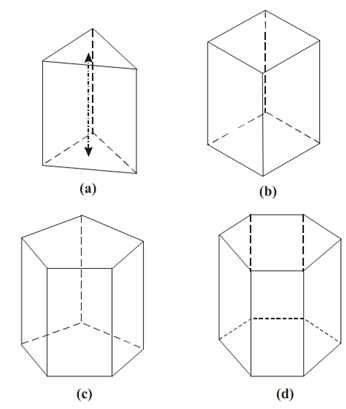## Prism Assignment Help

Prism:

A prism is a polyhedron containing two equal ends or bases parallel to each other. These two bases are joined with faces that are rectangles. The line joining the centers of the base is named as the axis of the prism.

However right and regular prism has its axis perpendicular to the bases and all its faces are rectangles. So according to the shape of base the prism might be triangular in diagram (a), square in diagram (b), pentagonal in diagram (c), or hexagonal in diagram (d).